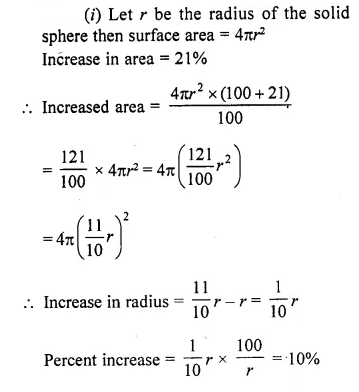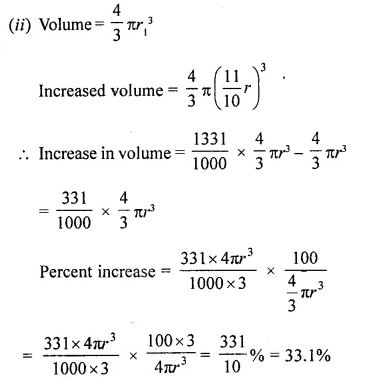# Selina Concise Mathematics Class 10 ICSE Solutions Chapter 20 Cylinder, Cone and Sphere Ex 20C

## Selina Concise Mathematics Class 10 ICSE Solutions Chapter 20 Cylinder, Cone and Sphere (Surface Area and Volume) Ex 20C

These Solutions are part of Selina Concise Mathematics Class 10 ICSE Solutions. Here we have given Selina Concise Mathematics Class 10 ICSE Solutions Chapter 21 Trigonometrical Identities Ex 21C

Other Exercises

Question 1.
The surface area of a sphere is 2464 cm2, find its volume.
Solution:
Surface area of sphere = 2464 cm2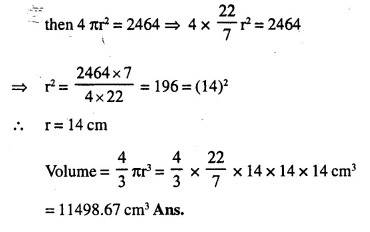Question 2.
The volume of a sphere is 38808 cm3; find its diameter and the surface area.
Solution:
Volume of sphere = 38808 cm3
Let radius of shpere = r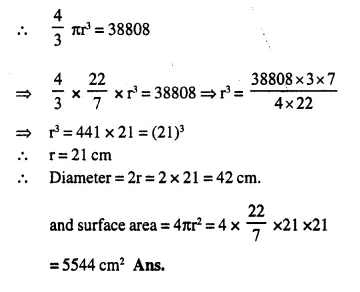Question 3.
A spherical ball of lead has been melted and made into identical smaller balls with radius equal to half the radius of the original one. How many such balls can be made ?
Solution:
Let the radius of spherical ball = r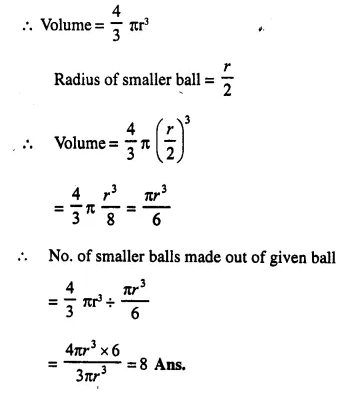Question 4.
How many balls each of radius 1 cm can be made by melting a bigger ball whose diameter is 8 cm.
Solution: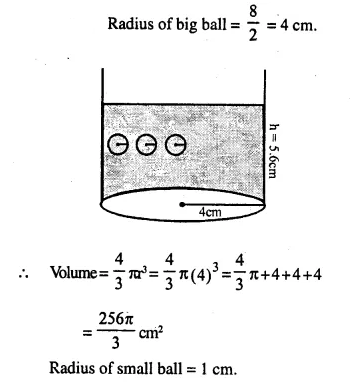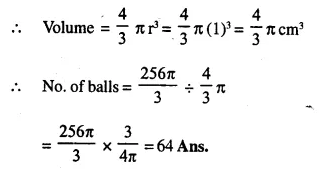Question 5.
Eight Metallic sphere; each of radius 2 mm, are melted and cast into a single sphere. Calculate the radius of the new sphere.
Solution:
Radius of metallic sphere = 2mm = $$\frac { 1 }{ 5 }$$ cm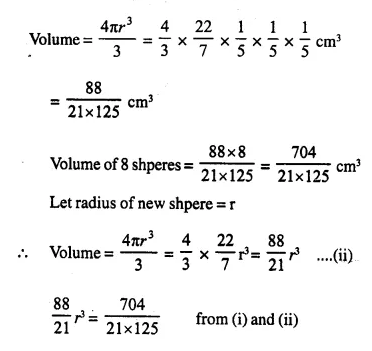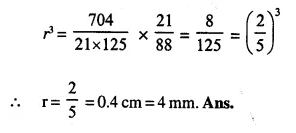Question 6.
The volume of one sphere is 27 times that of another sphere. Calculate the ratio of their:
(ii) surface areas.
Solution:
Volume of first sphere = 27 x volume of second sphere.
Let radius of first sphere = r1
and radius of second sphere = r2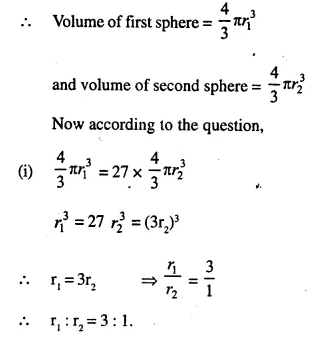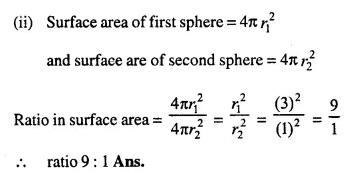Question 7.
If the number of square centimetres on the surface of a sphere is equal to the number of cubic centimetres in its volume, what is the diameter of the sphere ?
Solution: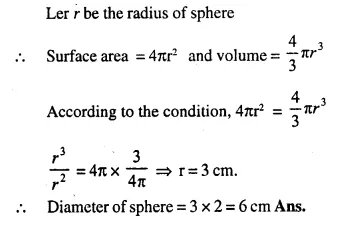Question 8.
A solid metal sphere is cut through its centre into 2 equal parts. If the diameter of the sphere is 3$$\frac { 1 }{ 2 }$$ cm, find the total surface area of each part correct to two decimal places.
Solution:
A solid sphere is cut into two equal hemispheres.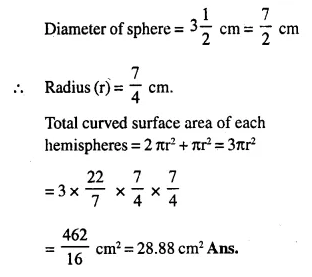Question 9.
The internal and external diameters of a hol­low spectively. Find:
(i) internal curved suface area,
(ii) external curved surface area,
(iii) total surface area,
(iv) volume of material of the vessel.
Solution:
Internal diameter of hollow hemispher = 21cm
and external diameter = 28 cm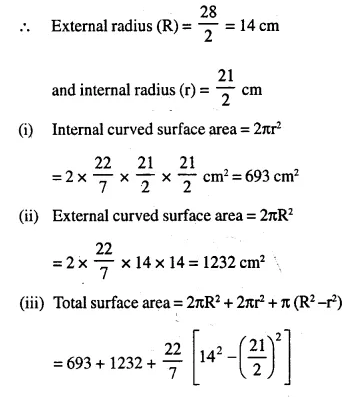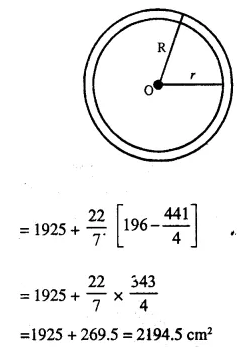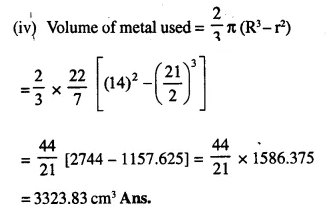Question 10.
A solid sphere and a solid hemi-sphere have the same total surface area. Find the ratio between their volumes.
Solution:
Let radius of a sphere = R
∴  Surface area = 4πR2
and radius of hemi-sphere = r
∴ Surface area = 3πr2
∵ Their surface area are equal
4πR2 = 3πr2 ⇒ 4R2 = 3r2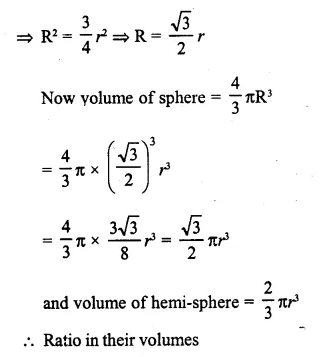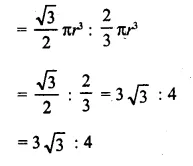Question 11.
Metallic spheres of radii 6 cm, 8 cm and 10 cm respectively are melted and recasted into a single solid sphere. Taking π = 3.1, find the surface area of solid sphere formed.
Solution:
Radius of first sphere (r1) = 6 cm
Radius of second sphere (r2) = 8 cm
Radius of third sphere (r3) = 10 cm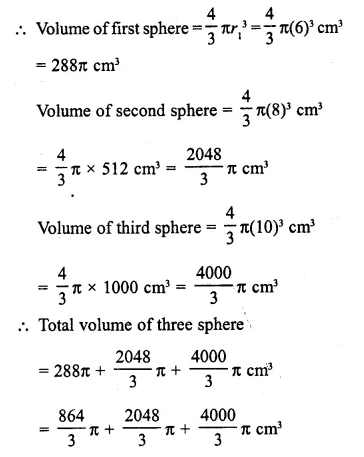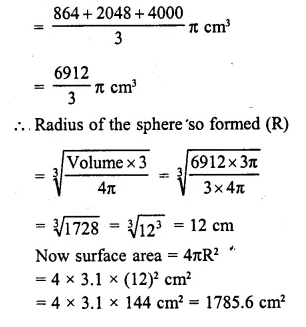Question 12.
The surface area of a solid sphere is increased by 21% without changing its shape. Find the percentage increase in its: